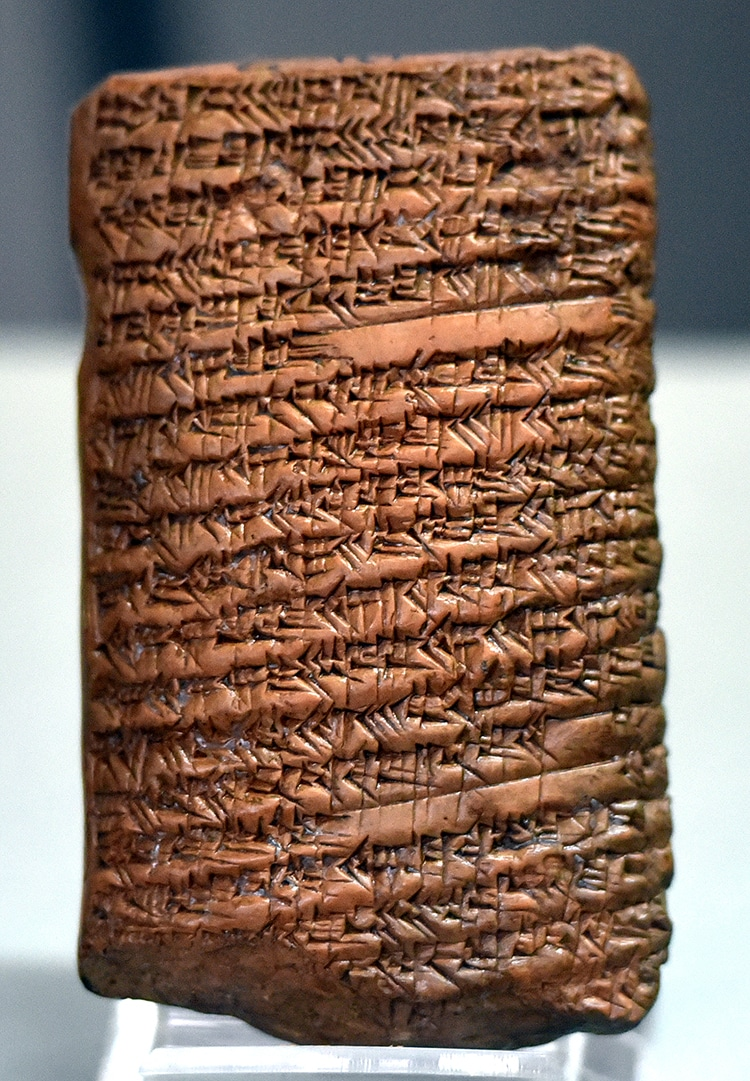# Ancient Babylonian Tablet Uses Pythagorean Theorem 1,000 Years Before Pythagoras Was BornThe ancient Babylonian tablet in cuneiform describes the pythagorean theorem. (Photo: Osama Shukir Muhammed Amin FRCP(Glasg) via Wikimedia Commons, CC BY-SA 4.0 DEED)

The Pythagorean Theorem is fundamental to geometry. Named after the ancient Greek philosopher and mathematician Pythagoras, the simple equation (c^2 = a^2 + b^2, where c is the hypotenuse) helps determine the missing value of a right triangle when you know the other two sides. Its application has been vital to technical endeavors like engineering and design for thousands of years. However, despite the name of the theorem, the numerical relationship of the sides of a triangle was known long before Pythagoras was born around 570 BCE. Ancient clay tablets suggest that the ancient Babylonians knew of this relationship as early as 2000 BCE.

The tablet above is a clay tablet discovered in Iraq and covered in cuneiform writing. It dates to sometime between 2000 and 1500 BCE. It was likely used as a teaching tool, because it describes how the “Pythagorean” theorem can be used to find the length of the diagonal of a rectangle. (Hint: A rectangle is two right triangles of equal side put together at the rectangle's diagonal, which is also the triangles' hypotenuses.) This ancient text is not the only evidence that the Babylonian's used this equation. Drawings on other tablets depict triangles which look amazingly similar to proofs of the theorem (see below).

So why does Pythagoras get all the credit, when Babylonians and likely ancient Egyptians and Indians knew the relationship between the sides of a right triangle? It is possible the heavy influence of the Pythagorean school, disciples of the man himself who passed down his teachings, may have influenced this. It is also true that much of ancient historical studies has been deeply Eurocentric. However, it is always important to remember the ancient mathematical traditions of non-European cultures and the interchange of ideas that happened over the millennia.

## Clay tablets from ancient Babylon use the Pythagorean Theorem long before the ancient Greek mathematician Pythagoras was born.A Babylonian tablet using the Pythagorean Theorem to calculate a problem around 1,900 BCE. It reads: 4 is the length and 5 is the diagonal. What is the breadth? Its size is not known. 4 times 4 is 16. And 5 times 5 is 25. You take 16 from 25 and there remains 9. What times what shall I take in order to get 9? 3 times 3 is 9. 3 is the breadth.(Photo: Fig. 3, Ratner)

## Related Articles:

NASA Mathematician Katherine Johnson Is Honored With a New Spacecraft Named After Her

Helpful Infographics Visualize Complex Branches of Math and Science

Who Was Pierre de Fermat? The Mathematician Who Left Behind a Mysterious “Last Theorem”

Who Was Isaac Newton? Get to Know the Alchemist, Physicist, and Mathematician